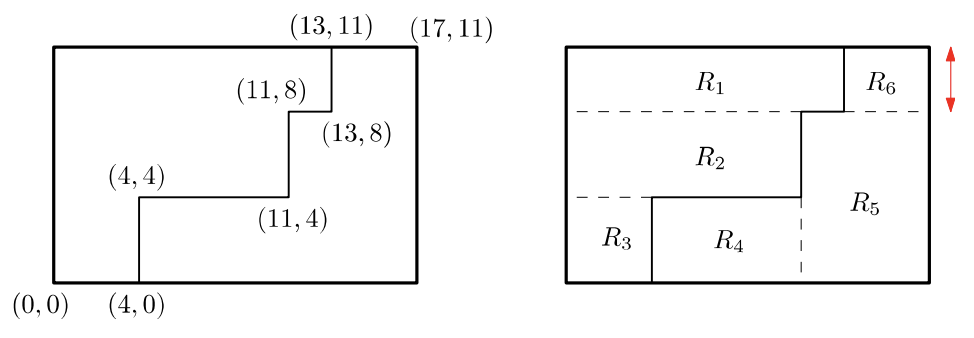시간 제한메모리 제한제출정답맞힌 사람정답 비율
0.5 초 512 MB0000.000%

## 문제

A rectangular tile fell to the ground and broke into two pieces. Fortunately, the tile was broken along a rectilinear curve monotone to the sides of the tile as shown in the left figure below. A rectilinear curve is composed of line segments. A rectilinear curve R is monotone to a straight line or line segment L if every straight line orthogonal to L intersects R at most once.

Since the tiles must be rectangular, we decided to cut each piece further using a cutter into rectangular tiles along lines through the sides of the piece. We call such a cut a rectangular cut of the pieces. Every rectangular cut partitions the two pieces into rectangles. Among all possible rectangular cuts of the pieces, we want to find a rectangular cut that maximizes the minimum side-length of the resulting rectangles of the pieces.For example, the left figure above shows an axis-aligned rectangle of side lengths 17 and 11. It is broken into two pieces along the monotone rectilinear curve connecting points (4,0), (4,4), (11,4), (11,8), (13,8), (13,11) by line segments in order. In a rectangular cut, you are allowed to cut each piece along the horizontal or vertical line or both from a corner vertex at (4,4), (11,4), (11,8) or (13,8) . The right figure shows a rectangular cut. For the left piece, we choose horizontal lines at (4,4) and (11,8). For the right piece, we choose a horizontal line at (13,8) and a vertical line at (11,4). Thus, this rectangular cut partitions the two pieces into 6 rectangles R1, R2, … , R6. Since R1 and R6 have the minimum side-length 3 among the 6 rectangles, this rectangular cut has the minimum side-length 3. Observe that this rectangular cut maximizes the minimum side-length among all possible rectangular cuts on the broken pieces.

Given an axis-aligned rectangle and a list of n points representing the monotone rectilinear curve breaking the rectangle into two pieces, write a program to output the maximum of the minimum side-lengths among all possible rectangular cuts.

## 입력

Your program is to read from standard input. The input starts with a line containing two integers, a and b (1 ≤ a ≤ 100,000, 1 ≤ b ≤ 100,000), where a and b are the x-coordinate and the y-coordinate of the topright corner of the axis-aligned rectangle with bottom-left corner at (0,0). There is no input with a = b = 1. The next line contains one integer n (2 ≤ n ≤ 100,000), where n is the number of points of the monotone rectilinear curve breaking the rectangle into two pieces. In the following n lines, the i-th line contains two integers, c and d (0 ≤ ca, 0 ≤ db), where c and d are the x-coordinate and the y-coordinate of the i-th point along the monotone rectilinear curve. You may assume that all the points are distinct.

## 출력

Your program is to write to standard output. Print exactly one line. The line should contain the maximum of the minimum side-lengths among all possible rectangular cuts.

## 예제 입력 1

3 4
2
1 0
1 4


## 예제 출력 1

1


## 예제 입력 2

4 5
4
4 2
2 2
2 3
0 3


## 예제 출력 2

2


## 예제 입력 3

17 11
6
4 0
4 4
11 4
11 8
13 8
13 11


## 예제 출력 3

3